esson: Factoring Polynomials
 The Multiplication Property of Zero This method requires knowledge of a property that involves the number zero. To elicit this property, consider this equation.What m-values and n-values make this equation true?     According to the multiplication property of zero, the equation is true when either...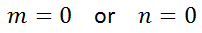We will use this property to solve quadratic equations within the examples below. Example 1 Here is the first quadratic equation we will solve.Since one side of the equation is equal to zero, the first step is to factor the polynomial.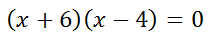Next, we use The Multiplication Property of Zero. This means we set the factors equal to zero.Solving each of these linear equations separately, we get…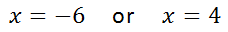If you would like to view our video on this topic, use the link below.ideo: Solve Quadratic Equations by Factoring     If you would like to test your skills with our quizmaster on this topic, use the link below.uiz: Solving Quadratic Equations: Factoring Example 2 Here is the second quadratic equation we will solve.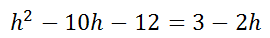Since the equation does not have a zero on one side, we cannot utilize The Multiplication Property of Zero. So, we need to get a zero by subtracting 3 from both sides and adding 2h to both sides. Doing so gives us this new equation.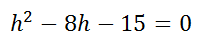Now we need to factor the polynomial.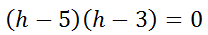Next, we set the factors equal to zero.Solving these linear equations yields…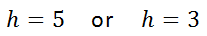If you would like to view our video on this topic, use the link below.ideo: Solve Quadratic Equations by Factoring     If you would like to test your skills with our quizmaster on this topic, use the link below.uiz: Solving Quadratic Equations: Factoring Instructional Videos Try this instructional video to learn how to solve quadratic equations by factoring.ideo: Solving Quadratic Equations: Factoring Interactive Quizmasters Try this interactive quizmaster to determine if you understand how to solve quadratic equations by factoring.uiz: Solving Quadratic Equations: Factoring Related Lessons Try these related lessons.esson: Factoring Polynomialsesson: Operations on Polynomialsesson: Solving Quadratic Equations